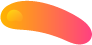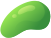##### Thermal and Statistical Physics

1) Consider a particle that has only three energy levels, E1 = 0, E2 =  , and E3 = 10 . The particle is in equilibrium at temperature T.
a) Write out the partition function for this particle.
b) Find the probability that the particle is in the level with energy E3.
c) If the system contains N such particles (non-interacting), find N3, the number of particles in the level with energy E3.
d) Find the temperature Tc below which there are no particles in the level with energy E3 (i.e., N3 < 1); you may assume N >>1.
e) Find the mean energy per particle.
f) Find the heat capacity Cv of the system.
g) Find the behavior of Cv at high (kT >> ) and low (kT << ) temperatures.
h) Sketch Cv vs. T.